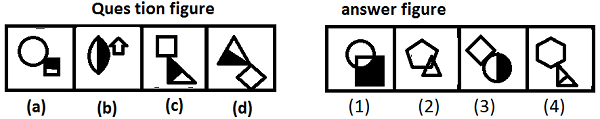•••••••••••••••••••••••••••••••••••••••••••••••••••••••••••••••••••••••••••••••••••• Home /Exam Details (QP Included) / Main Exam / Compulsory Papers / Paper VI : Arithmetic and Test of Reasoning / Reasoning Notes – Figure Series – For W.B.C.S. Examination.
•Posted on May 20th, 2019 in Paper VI : Arithmetic and Test of Reasoning
Tags:

## Reasoning Notes – Figure Series – For W.B.C.S. Examination.

Figure series problems are generally asked in competitive exams to test reasoning ability of candidates. It needs ability of a candidate to take quick decisions, fast problem solving capability, good comparing ability and quickness in finding reason behind various problems. It tests ability of candidate to imagine quick and proper solution. The figure on question may contain various geometrical figures, numbers, symbols or other pictures. Problems on figure series may be of different types.Continue Reading Reasoning Notes – Figure Series – For W.B.C.S. Examination.

Various types of questions asked are,

Example-1

While dealing with these types of figures, a candidate has to find out the sequence of series in which each figure is changing one by one or has to find the relation between each of them.

For example let’s find the figure that will be continue the series given below.

Example − To find the figure which will continue a series of figure?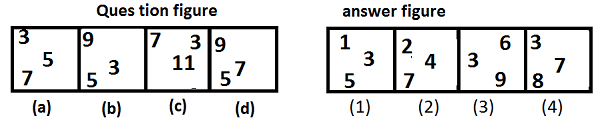Explanation −

From above figure, it is clear that question figure consist of various set of numbers in each box which are odd numbers.

Example-2

Candidate may be asked to choose the correct figure from given options that will replace question mark on the question figure. These types of problem generally consist of series of figures which are changing their shape, look, or position according to a certain sequence. A candidate has to compare figures given and find out sequence of figure then to choose correct figure from options given which will follow the sequence. It can be demonstrated by example given below.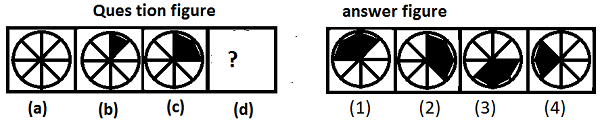Example − Find the figure that will replace the question mark.

Explanation −

The sequence of this series is that the circle has been divided into various sectors which are getting shaded in clockwise direction by adding a cord each time.

Example-3

Sometimes two co-related figures will be given and question will be asked to find out the figure from given options that is in the sequence with a 3rd figure. The candidate has to know sequence of first two figures in which they are related to each other and choose one of the options that is related to 3rd figure in same way as that of first pair of figures. Following example will illustrate it more clearly.

Example − Which figure can be placed in place of question mark?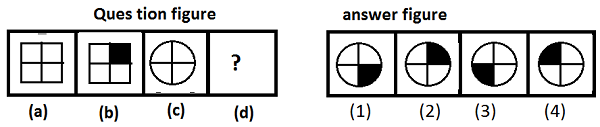Explanation − Figure (a) and (b) are related to each other by getting shade of upper right side quarter therefore in this way figure (2) will make pair with figure (c).

Example-4

Questions may be given that will consist of a core figure and would be changing in each step according to certain sequence. Questions will be asked to find the figure that would be different from others that is violating the sequence of series.

Example − Find out the figure which is different from others in figure below.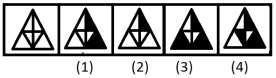Explanation − In each step, two spaces of the main figure is being shaded and one space is erased in next step this sequence repeated throughout the series, according to this sequence 4th figure must have two shaded figures but not so, therefore is the answer figure.

Example-5

Sometimes a series of similar figures would be given and candidate will be asked to find similar figure from option given as illustrated by example below.

Example − Find the similar figure as that of the question figure.